# Ohio Open Ed Collaborative Calculus

This content was created as part of an Ohio Department of Higher Education Innovation Grant to create Open Educational Resources for high enrollment courses. A team of faculty content collaborators, a librarian, and a faculty review team worked together to curate this content and assure that it meets the Transfer Assurance Guidelines for this course. The Calculus I and Calculus II Courses are designed to help the instructor teach all of the objectives of the course and can be used as a whole or in pieces or modules. The full courses are entitled Calculus I Course Content and Calculus II Course Content. This work was completed and the courses were posted in February 2019. Please visit ohioopened.org for more information about this initiative.

96 affiliated resources
View
Selected filters:Rating

The Calculus I course was developed through the Ohio Department of Higher Education OER Innovation Grant. This work was completed and the course was posted in February 2019. The course is part of the Ohio Transfer Module and is also named TMM005. For more information about credit transfer between Ohio colleges and universities, please visit: www.ohiohighered.org/transfer.Team LeadJim Fowler                                         Ohio State UniversityRita Ralph                                         Columbus State Community CollegeContent ContributorsNela Lakos                                       Ohio State UniversityBart Snapp                                       Ohio State UniversityJames Talamo                                  Ohio State UniversityXiang Yan                                         Edison State Community CollegeLibrarianDaniel Dotson                                    Ohio State University                     Review TeamThomas Needham                             Ohio State UniversityCarl Stitz                                            Lakeland Community CollegeSara Rollo                                          North Central State College

Subject:
Mathematics
Calculus
Material Type:
Full Course
Provider:
Ohio Open Ed Collaborative
09/26/2018Conditions of Use:
Remix and Share
Rating

After completing this section, students should be able to do the following.Compute average velocity.Approximate instantaneous velocity.Compare average and instantaneous velocity.Compute instantaneous velocity.

Subject:
Calculus
Material Type:
Module
Provider:
Ohio Open Ed Collaborative
08/15/2019Conditions of Use:
Remix and Share
Rating

After completing this section, students should be able to do the following.Define an antiderivative.Compute basic antiderivatives.Compare and contrast finding derivatives and finding antiderivatives.Define initial value problems.Solve basic initial value problems.Use antiderivatives to solve simple word problems.Discuss the meaning of antiderivatives of the velocity and acceleration.

Subject:
Calculus
Material Type:
Module
Provider:
Ohio Open Ed Collaborative
08/15/2019Conditions of Use:
Remix and Share
Rating

After completing this section, students should be able to do the following.Interpert the product of rate and time as area.Approximate position from velocity.Recognize Riemann sums.

Subject:
Calculus
Material Type:
Module
Provider:
Ohio Open Ed Collaborative
08/15/2019Conditions of Use:
Remix and Share
Rating

After completing this section, students should be able to do the following.Given a velocity function, calculate displacement and distance traveled.Given a velocity function, find the position function.Given an acceleration function, find the velocity function.Understand the difference between displacement and distance traveled.Understand the relationship between position, velocity and acceleration.Calculate the change in the amount.Compute the average value of the function on an interval.Understand that the average value of the function on an interval is attained by the function on that interval.

Subject:
Calculus
Material Type:
Module
Provider:
Ohio Open Ed Collaborative
08/15/2019Conditions of Use:
Remix and Share
Rating

After completing this section, students should be able to do the following.Identify word problems as related rates problems.Translate word problems into mathematical equations.Solve related rates word problems.

Subject:
Calculus
Material Type:
Module
Provider:
Ohio Open Ed Collaborative
08/15/2019Conditions of Use:
Remix and Share
Rating

After completing this section, students should be able to do the following.Express the sum of n terms using sigma notation.Apply the properties of sums when working with sums in sigma notation.Understand the relationship between area under a curve and sums of areas of rectangles.Approximate area of the region under a curve.Compute left, right, and midpoint Riemann sums with 10 or fewer rectangles.Understand how Riemann sums with n rectangles are computed and how the exact value of the area is obtained by taking the limit as n→∞n→∞ .

Subject:
Calculus
Material Type:
Module
Provider:
Ohio Open Ed Collaborative
08/15/2019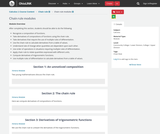Conditions of Use:
Remix and Share
Rating

After completing this section, students should be able to do the following.Recognize a composition of functions.Take derivatives of compositions of functions using the chain rule.Take derivatives that require the use of multiple rules of differentiation.Use the chain rule to calculate derivatives from a table of values.Understand rate of change when quantities are dependent upon each other.Use order of operations in situations requiring multiple rules of differentiation.Apply chain rule to relate quantities expressed with different units.Compute derivatives of trigonometric functions.Use multiple rules of differentiation to calculate derivatives from a table of values.

Subject:
Calculus
Material Type:
Module
Provider:
Ohio Open Ed Collaborative
08/15/2019Conditions of Use:
Remix and Share
Rating

After completing this section, students should be able to do the following.Find the intervals where a function is increasing or decreasing.Find the intervals where a function is concave up or down.Determine how the graph of a function looks without using a calculator.

Subject:
Calculus
Material Type:
Module
Provider:
Ohio Open Ed Collaborative
08/15/2019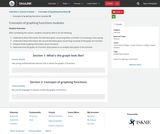Conditions of Use:
Remix and Share
Rating

After completing this section, students should be able to do the following.Understand what information the derivative gives concerning when a function is increasing or decreasing.Understand what information the second derivative gives concerning concavity of the graph of a function.Interpret limits as giving information about functions.Determine how the graph of a function looks based on an analytic description of the function.

Subject:
Calculus
Material Type:
Module
Provider:
Ohio Open Ed Collaborative
07/02/2019Conditions of Use:
Remix and Share
Rating

After completing this section, students should be able to do the following.Identify where a function is, and is not, continuous.Understand the connection between continuity of a function and the value of a limit.Make a piecewise function continuous.State the Intermediate Value Theorem including hypotheses.Determine if the Intermediate Value Theorem applies.Sketch pictures indicating why the Intermediate Value Theorem is true, and why all hypotheses are necessary.Explain why certain points exist using the Intermediate Value Theorem.

Subject:
Calculus
Material Type:
Module
Provider:
Ohio Open Ed Collaborative
08/15/2019Conditions of Use:
Remix and Share
Rating

After completing this section, students should be able to do the following.Use integral notation for both antiderivatives and definite integrals.Compute definite integrals using geometry.Compute definite integrals using the properties of integrals.Justify the properties of definite integrals using algebra or geometry.Understand how Riemann sums are used to find exact area.Define net area.Approximate net area.Split the area under a curve into several pieces to aid with calculations.Use symmetry to calculate definite integrals.Explain geometrically why symmetry of a function simplifies calculation of some definite integrals.

Subject:
Calculus
Material Type:
Module
Provider:
Ohio Open Ed Collaborative
08/15/2019Conditions of Use:
Remix and Share
Rating

After completing this section, students should be able to do the following.Use limits to find the slope of the tangent line at a point.Understand the definition of the derivative at a point.Compute the derivative of a function at a point.Estimate the slope of the tangent line graphically.Write the equation of the tangent line to a graph of a function at a given point.Recognize and distinguish between secant and tangent lines.Recognize the the tangent line as a local approximation for a differentiable function near a point.

Subject:
Calculus
Material Type:
Module
Provider:
Ohio Open Ed Collaborative
08/15/2019Conditions of Use:
Remix and Share
Rating

After completing this section, students should be able to do the following.Understand the derivative as a function related to the original definition of a function.Find the derivative function using the limit definition.Relate the derivative function to the derivative at a point.Explain the relationship between differentiability and continuity.Relate the graph of the function to the graph of its derivative.Determine whether a piecewise function is differentiable.

Subject:
Calculus
Material Type:
Module
Provider:
Ohio Open Ed Collaborative
08/15/2019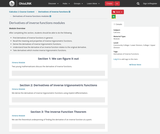Conditions of Use:
Remix and Share
Rating

After completing this section, students should be able to do the following.Find derivatives of inverse functions in general.Recall the meaning and properties of inverse trigonometric functions.Derive the derivatives of inverse trigonometric functions.Understand how the derivative of an inverse function relates to the original derivative.Take derivatives which involve inverse trigonometric functions.

Subject:
Calculus
Material Type:
Module
Provider:
Ohio Open Ed Collaborative
08/15/2019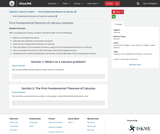Conditions of Use:
Remix and Share
Rating

After completing this section, students should be able to do the following.Define accumulation functions.Calculate and evaluate accumulation functions.State the First Fundamental Theorem of Calculus.Take derivatives of accumulation functions using the First Fundamental Theorem of Calculus.Use accumulation functions to find information about the original function.Understand the relationship between the function and the derivative of its accumulation function.

Subject:
Calculus
Material Type:
Module
Provider:
Ohio Open Ed Collaborative
08/15/2019Conditions of Use:
Remix and Share
Rating

After completing this section, students should be able to do the following.Use the first derivative to determine whether a function is increasing or decreasing.Define higher order derivatives.Compare differing notations for higher order derivatives.Identify the relationships between the function and its first and second derivatives.Sketch a graph of the second derivative, given the original function.Sketch a graph of the original function, given the graph of its first or second derivative.Sketch a graph of a function satisfying certain constraints on its higher-order derivatives.State the relationship between concavity and the second derivative.Interpret the second derivative of a position function as acceleration.Calculate higher order derivatives.Given the graph of a position function, find time intervals where the velocity is positive/negativeGiven the graph of a position function, find time intervals where the velocity is increasing/decreasing

Subject:
Calculus
Material Type:
Module
Provider:
Ohio Open Ed Collaborative
08/15/2019Conditions of Use:
Remix and Share
Rating

After completing this section, students should be able to do the following.Implicitly differentiate expression.Find the equation of the tangent line for curves that are not plots of functions.Understand how changing the variable changes how we take the derivative.Understand the derivatives of functions that are not defined explicitly in terms of an independent variable .Explain the formula for the derivative of the natural log function.Calculate derivatives of logs in any base.Calculate the derivative of an exponential function, axax, where a>0a>0.

Subject:
Calculus
Material Type:
Module
Provider:
Ohio Open Ed Collaborative
08/15/2019Conditions of Use:
Remix and Share
Rating

After completing this section, students should be able to do the following.Understand what is meant by the form of a limit.Calculate limits of the form zero over zero.Calculate limits of the form nonzero number over zero.Identify determinate and indeterminate forms.Distinguish between determinate and indeterminate forms.Discuss why infinity is not a number.

Subject:
Calculus
Material Type:
Module
Provider:
Ohio Open Ed Collaborative
08/15/2019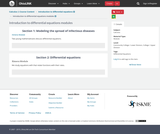Conditions of Use:
Remix and Share
Rating

Subject:
Calculus
Material Type:
Module
Provider:
Ohio Open Ed Collaborative
08/15/2019Conditions of Use:
Remix and Share
Rating

After completing this section, students should be able to do the following.Calculate limits using the limit laws.Calculate limits by replacing a function with a continuous function that has the same limit.Understand the Squeeze Theorem and how it can be used to find limit values.Calculate limits using the Squeeze Theorem.

Subject:
Calculus
Material Type:
Module
Provider:
Ohio Open Ed Collaborative
08/15/2019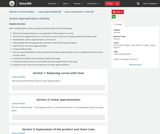Conditions of Use:
Remix and Share
Rating

After completing this section, students should be able to do the following.Define linear approximation as an application of the tangent to a curve.Find the linear approximation to a function at a point and use it to approximate the function value.Identify when a linear approximation can be used.Label a graph with the appropriate quantities used in linear approximation.Find the error of a linear approximation.Compute differentials.Use the second derivative to discuss whether the linear approximation over or underestimates the actual function value.Contrast the notation and meaning of dydy versus ΔyΔy.Understand that the error shrinks faster than the displacement in the input.Justify the chain rule via the composition of linear approximations.

Subject:
Calculus
Material Type:
Module
Provider:
Ohio Open Ed Collaborative
08/15/2019Conditions of Use:
Remix and Share
Rating

After completing this section, students should be able to do the following.Identify situations where logs can be used to help find derivatives.Use logarithmic differentiation to simplify taking derivatives.Take derivatives of functions raised to functions.Recognize the difference between a variable in the base and a variable in the exponent.

Subject:
Calculus
Material Type:
Module
Provider:
Ohio Open Ed Collaborative
08/15/2019Conditions of Use:
Remix and Share
Rating

After completing this section, students should be able to do the following.Define a critical point.Find critical points.Define local maximum and local minimum.Classify critical points.State the First Derivative Test.Apply the First Derivative Test.Define inflection points.Find inflection points.State the Second Derivative Test.Apply the Second Derivative Test.

Subject:
Calculus
Material Type:
Module
Provider:
Ohio Open Ed Collaborative